# ML Aggarwal Class 10 Solutions for ICSE Maths Chapter 21 Measures of Central Tendency Ex 21.5

## ML Aggarwal Class 10 Solutions for ICSE Maths Chapter 21 Measures of Central Tendency Ex 21.5

These Solutions are part of ML Aggarwal Class 10 Solutions for ICSE Maths. Here we have given ML Aggarwal Class 10 Solutions for ICSE Maths Chapter 21 Measures of Central Tendency Ex 21.5

More Exercises

Question 1.
Draw an ogive for the following frequency distribution: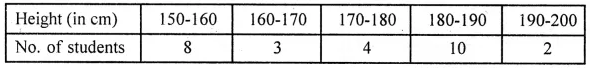Solution: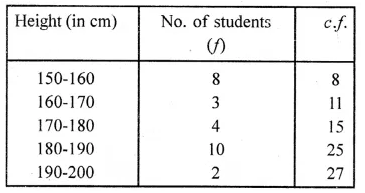Plot the points (160, 8), (170, 11), (180, 15), (190, 25) and (200, 27)
on the graph and join them with the free hand. We get an ogive as shown: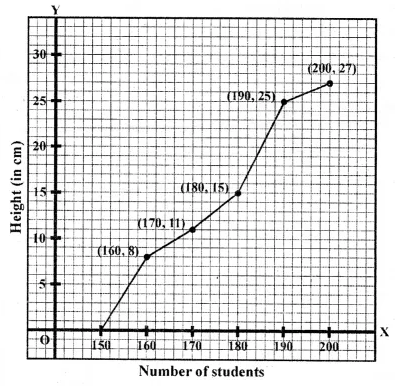Question 2.
Draw an ogive for the following data: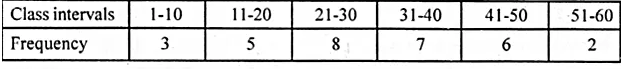Solution: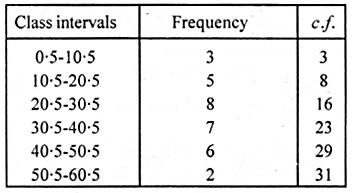Plot the points (10.5, 3), (20.5, 8), (30.5, 16), (40.5, 23),
(50.5, 29), (60.5, 31) on the graph and join them with a free hand,
we get an ogive as shown: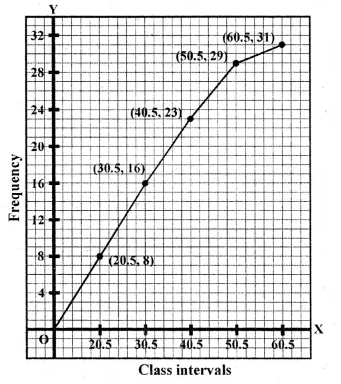Question 3.
Draw a cumulative frequency curve for the following data: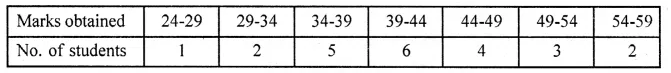Solution: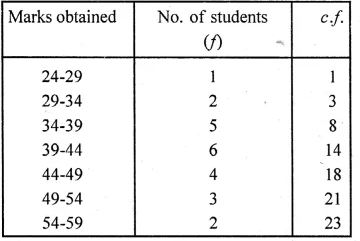Plot the points (29, 1), (34, 3), (39, 8), (44, 14), (49, 18),
(54, 21) and (59, 23) on the graph and join them
with a free hand to get an ogive as shown: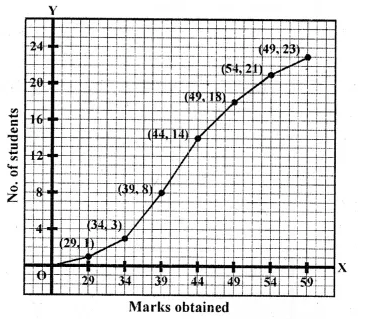We hope the ML Aggarwal Class 10 Solutions for ICSE Maths Chapter 21 Measures of Central Tendency Ex 21.5 help you. If you have any query regarding ML Aggarwal Class 10 Solutions for ICSE Maths Chapter 21 Measures of Central Tendency Ex 21.5, drop a comment below and we will get back to you at the earliest.